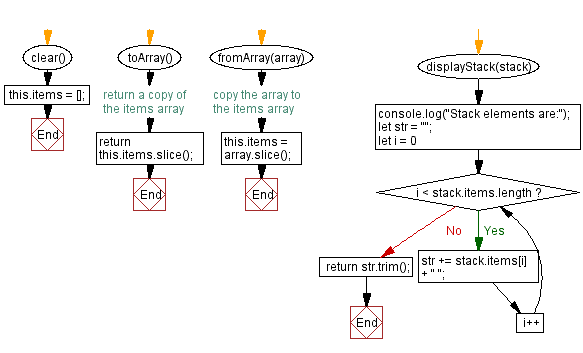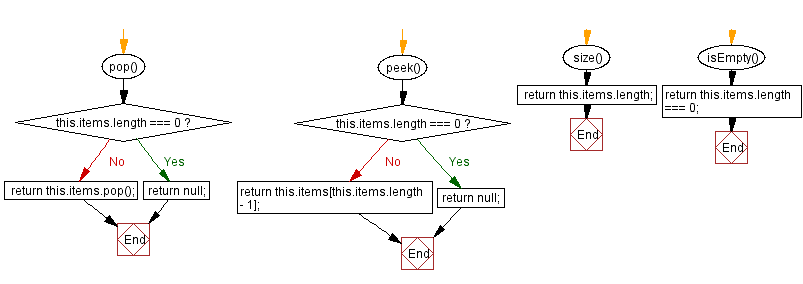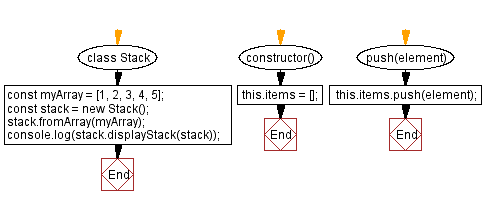# JavaScript Exercises: Create stacks from arrays

## JavaScript Stack: Exercise-23 with Solution

Write a JavaScript program that can create stacks from arrays.

In this exercise, we create an array myArray containing the values [1, 2, 3, 4, 5]. We then create a new stack object 'stack' using the Stack constructor function. We then call the from_Array method on 'stack', passing in myArray as the argument. This creates a new stack with the same values as myArray. Finally, we use the displayStack method to display the ‘stack’ elements

Sample Solution:

JavaScript Code:

``````class Stack {
constructor() {
this.items = [];
}
push(element) {
this.items.push(element);
}
pop() {
if (this.items.length === 0) {
return null;
}
return this.items.pop();
}

peek() {
if (this.items.length === 0) {
return null;
}
return this.items[this.items.length - 1];
}
size() {
return this.items.length;
}
isEmpty() {
return this.items.length === 0;
}
clear() {
this.items = [];
}
toArray() {
return this.items.slice(); // return a copy of the items array
}
fromArray(array) {
this.items = array.slice(); // copy the array to the items array
}
displayStack(stack) {
console.log("Stack elements are:");
let str = "";
for (let i = 0; i < stack.items.length; i++)
str += stack.items[i] + " ";
return str.trim();
}
}
const myArray = [1, 2, 3, 4, 5];
const stack = new Stack();
stack.fromArray(myArray);
console.log(stack.displayStack(stack));
```
```

Sample Output:

```Stack elements are:
1 2 3 4 5
```

Flowchart:Live Demo:

See the Pen javascript-stack-exercise-23 by w3resource (@w3resource) on CodePen.

Improve this sample solution and post your code through Disqus

Stack Previous: Convert a stack into an array.
Stack Exercises Next: Concatenates two stacks into a new stack.

What is the difficulty level of this exercise?

Test your Programming skills with w3resource's quiz.

﻿